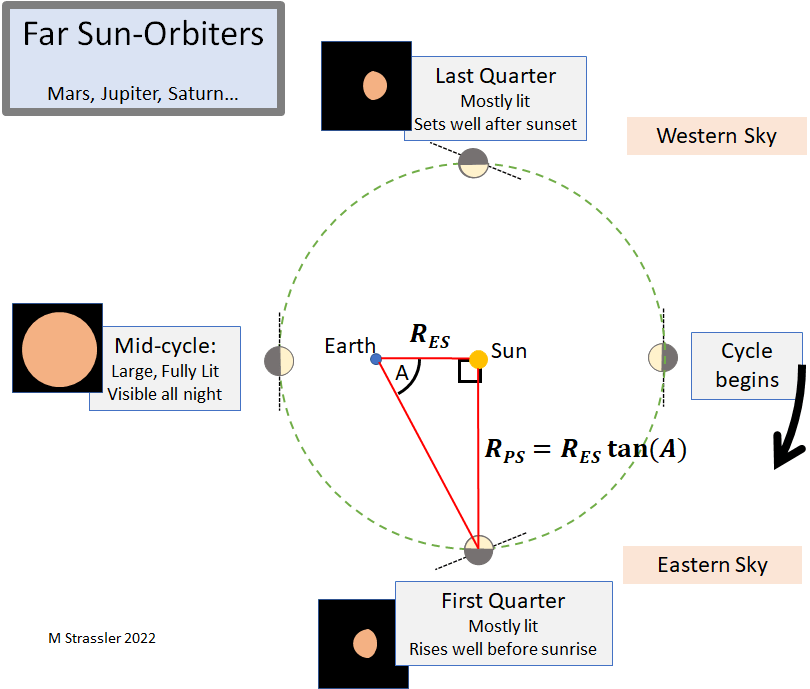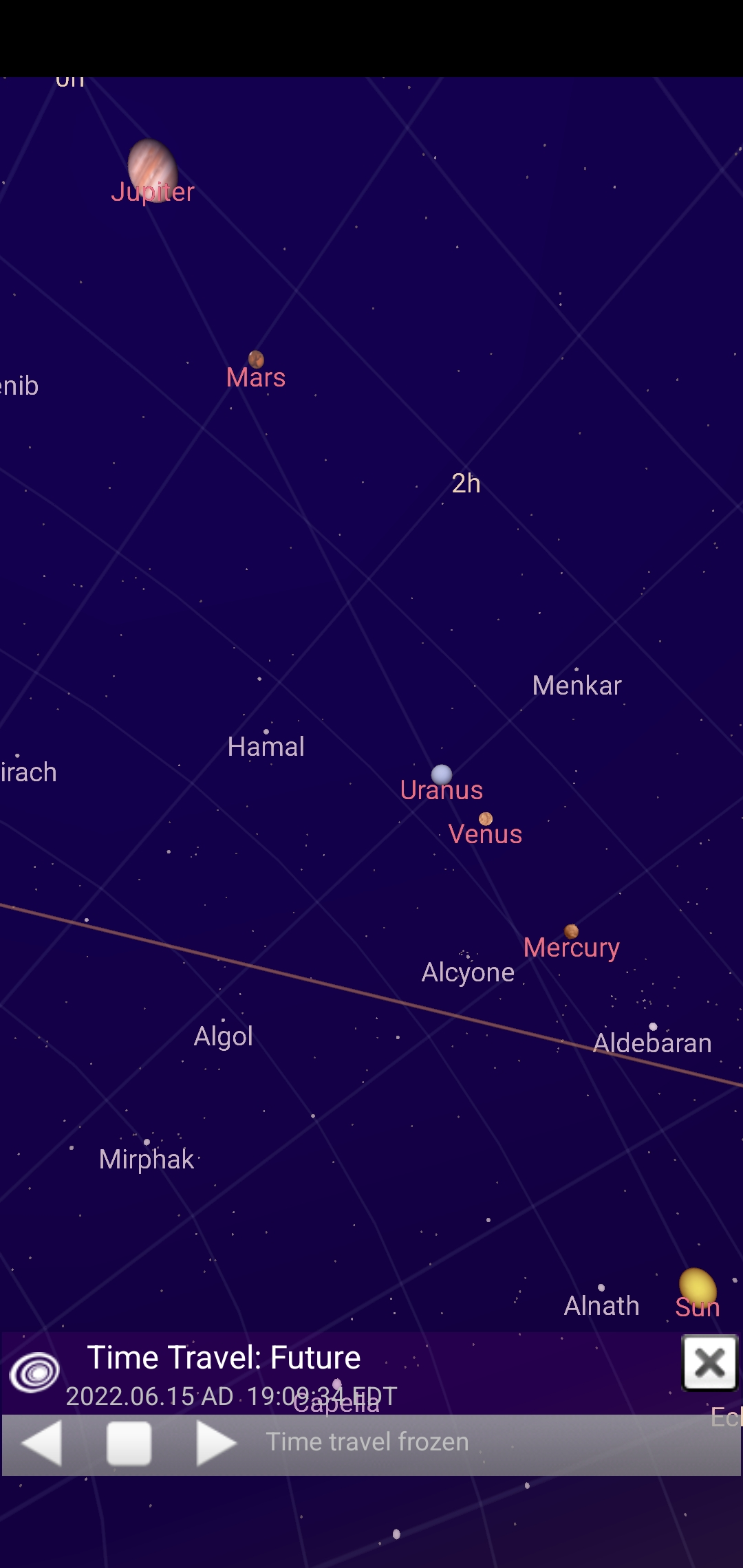# How to Estimate the Distance to the Outer Planets Yourself

Now, the last step in mapping out the other planets, before heading for more intriguing territory.

In a previous post I showed you how you can measure the distance between Venus and the Sun, RVS, relative to the distance between Earth and the Sun, RES. Under the assumption that Venus’s orbit around the Sun is circular (or nearly so), you can use the fact that when the angle between Venus and the Sun reaches its maximum (the moment of greatest elongation, and also approximately the moment when Venus appears half lit by the Sun), there’s a simple right-angle triangle in play. High school trigonometry then gives you the answer: RVS/RES ≈ 0.72 ≈ 1/√2. The same trick works for Mercury, which, like Venus, is a near Sun-orbiting planet, closer to the Sun than Earth.

But there’s no maximum angle for Mars, Jupiter, or the other far planets. These planets are further out than Earth and can even appear overhead at midnight, when they are 180 degrees away from the Sun. Fortunately there’s another right triangle we can use, again under the assumption of a (almost-)circular orbit, and that can give us a decent estimate.

### The Triangle for the Far Sun-Orbiting Planets

Let’s focus on Mars first, although the same technique will work on the outer planets. Mars has a cycle in which it disappears behind the Sun, from Earth’s perspective, on average every 780 days. (That start of the cycle is called “solar conjunction,” or just “conjunction” when the context is clear.) About half a cycle later, after on average 390 days, it is at “opposition”: closest to Earth, largest in a telescope, appearing overhead at midnight, and at its brightest. But if we wait only a quarter cycle, on average 195 days after conjunction, then the Mars-Sun line is at a 90 degree angle to the Earth-Sun line. That means that Mars, Earth and the Sun form a right-angle triangle with the right angle at the location of the Sun.

So on the day of first quarter we should measure the angle on the sky between Mars and the Sun. That’s the angle A on the figure below. Then the Mars-Sun distance RMS and the Earth-Sun distance RES are the two sides of a right-angle triangle. That means they are related by the tangent function:

• RMS/RES = tan A.For a planet whose distance RPS from the Sun is greater than Earth’s, an estimate of its distance compared to the Earth-Sun distance RES can be made using the fact that (were its orbit perfectly circular) it would make a right-angle triangle after the first or third quarter of its cycle, with the right angle located at the Sun. Sizes of Earth, Sun and planet not shown to scale.

Mars’ orbit is less circular than that of other planets excepting Mercury, and so each cycle is somewhat different. In the current cycle, the time between Mars’ last solar conjunction and its next opposition is 425 days, not 390. So should we take 195 days as first quarter, or 212 which is about half of 425? It will make some difference. We can try both ways and see how big the difference is. Sometimes, when you’re making estimates, you just have to accept that your answer won’t be super-precise. But at least it’s easy.

If we go for 212 days, it’s a measurement we can do on May 8th. That’s a particularly convenient date, because just by chance it’s also first quarter for Saturn, (whose last conjunction was on February 4 and whose next opposition is on August 14th.) If instead, for Mars, you go for the 195 day average after conjunction, that will get you to April 16th. Meanwhile, Jupiter’s most recent conjunction was on on March 5th, and with opposition September 26th, it will be at first quarter around June 15th. So if you’re willing to get up early, you can do all these estimates in the next three months. Or, as we did for Venus, you can use an astronomy app such as Sky Map.

As a preview, we can look ahead to the future, using Sky Map’s ability to show you what the sky looked like in the past and will look like in the future. Let’s see what it predicts you’ll observe. When the appointed date comes, you can of course check it for yourself; and you’ll find that Mars’ distance from the Sun is about 1.5 times larger than the Earth-Sun distance, while Jupiter’s is roughly 5 times larger and Saturn roughly 10 times larger.

### What Sky Map Predicts You’ll Find

Okay, for those who want to see how it works, here we go. I’m going to estimate the angles between Mars and the Sun, using the same method I used for Venus, with one caveat. The angles are big enough in this context that we need to be a bit more careful. The grid in the Sky Map pictures below actually represents coordinates on a sphere: the sphere of the sky. On a sphere, Pythagoras’ famous theorem, for a right triangle on a flat surface,

• a2 + b2 = c2 ,

is corrected to something a bit more complex. If you want to know how to get the angle between two points on the sky using this grid, you can read a description of the method here; it describes the coordinates that astronomers use, and gives a straightforward but ugly-looking trigonometric relation.Sky Map app prediction for May 9: the morning eastern sky, with the Sun at bottom right, Saturn at upper left and Mars below Saturn (indicating Saturn’s orbital radius is much larger than Mars’.)

The picture at right is Sky Map’s prediction for May 8th, a decent approximation to first quarter for both Mars and Saturn. For Mars, an estimate similar to what I did for Venus, but doing the trigonometry more carefully, I find about 60 degrees, which gives the Mars-Sun distance as 1.7 times the Earth-Sun distance (RMS/RES ≈ 1.7), and between Saturn and the Sun as about 84 degrees, giving its distance as RSS/RES ≈ 9.5 . But these estimates are not super-accurate, and for Saturn especially, that’s going to mean a substantial uncertainty in the planet-Sun distance. Once the angle is getting close to 90 degrees, even a shift of one degree can make a big difference on the estimated distance. For example, if we estimated the angle as 85 degrees, we’d get RSS/RES ≈ 11.4 the Earth-Sun distance, whereas 83 degrees would give RSS/RES ≈ 8.1. Even for Mars, shifting the angle by one degree up or down shifts RMS/RES up or down by about 0.07. Well, that’s life; we didn’t learn the size of the Earth or the distance to the Moon to high precision either.

Remember, we have another source of imperfection from the fact that since Mars is not on a circular orbit with constant speed, we don’t know exactly when the right triangle shown in the figure at the top actually occurs. If we took 190 days (1/4 of an average cycle) after the last conjunction, we’d be looking at Mars on April 16th, and if we play the same game with Sky Map (not shown, but you can try it), we find an angle closer to 55 degrees. That gives RMS/RES of only 1.4 times the Earth-Sun distance.

This might lead us to think the truth is somewhere in between these two estimates, and indeed it is: Mars distance from the Sun varies from 1.37 to 1.65 RES , a variation of almost 20%, with an average of 1.44 RES.Sky Map app prediction for June 15: the morning eastern sky, with the Sun at bottom right and Jupiter at upper left. Note the angle to Jupiter from the Sun lies between the angles seen in the previous figure for Saturn and Mars.

Jupiter’s most recent conjunction was on on March 5th, and with opposition September 26th, it will be at first quarter around June 15th, when Sky Map predicts the picture seen at right. That seems to give an angle of about 80 degrees, or RSS/RES ≈ 5.3, compared to the actual average of 5.2. But a change of the angle by just one degree would change 5.3 to 5.2 or 5.4, so again, a small discrepancy is not surprising.

The method used here won’t easily give precise measures of the orbital radii for Uranus and Neptune. You should however be able to work out that Uranus is further out than Saturn, and Neptune is the furthest of all.

So now you have it; between this post and the last one, you know how to map out the entire solar system, under the assumption that the planets’ orbits are near-circles. (We can check that assumption later.) But can we figure out how gravity works from this information, along with the lengths of the planets’ cycles? Yes We Can.

### 4 thoughts on “How to Estimate the Distance to the Outer Planets Yourself”

1.Hi Matt, small typo, you want to write 0.72\approx 1/\sqrt{2}. Long time since we last met! All the best!

•Thanks! Fixed.

2.This geometry should work for any roughly circular planetary orbit, no? For the Venus and Mercury, is it easier to pick the greatest elongation/half-lit point accurately, versus the quarter-cycle point? Or is the benefit in the former case that you don’t have to observe the planet for long enough to estimate its orbital period?

•Yes, the greatest elongation is easily identified and is especially good because it’s also insensitive to non-circularity. It’s interesting to see how Kepler approached the problem for the outer planets despite not assuming circularity; he assumed (with some evidence) that the orbits don’t precess and don’t change shape, and used that fact to map out the Earth’s orbit, and from there to map out the others’ . https://galileoandeinstein.phys.virginia.edu/1995/lectures/morekepl.html
http://ircamera.as.arizona.edu/astr_250/Lectures/LECTURE_02.HTM